Published

# MEGR 3171 IOT Project: Plant Moisture

Have you ever wondered if your plant needs more water? We will show you how to measure your soil for proper plant hydration treatment!

BeginnerWork in progress214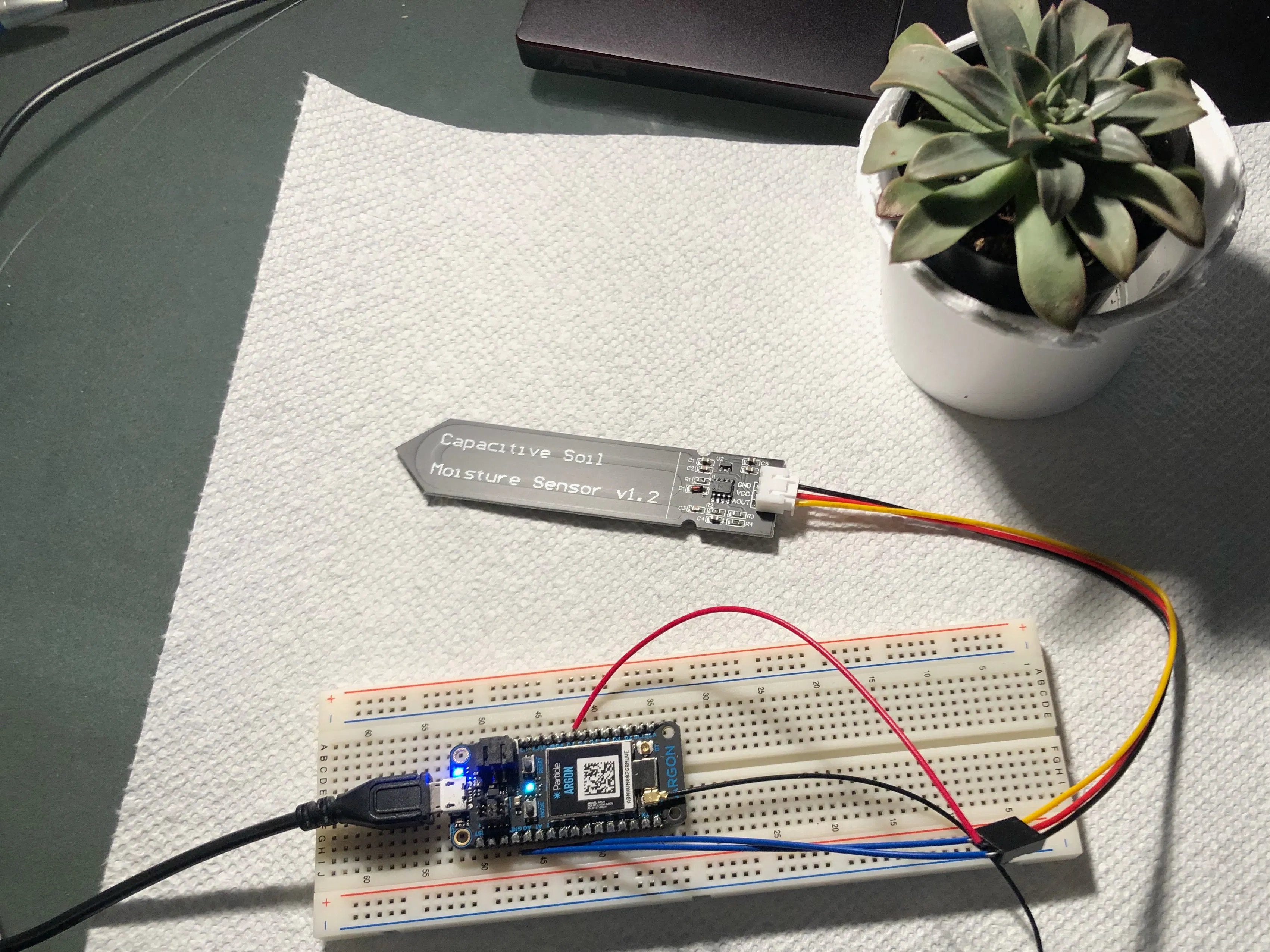## Things used in this project

### Hardware components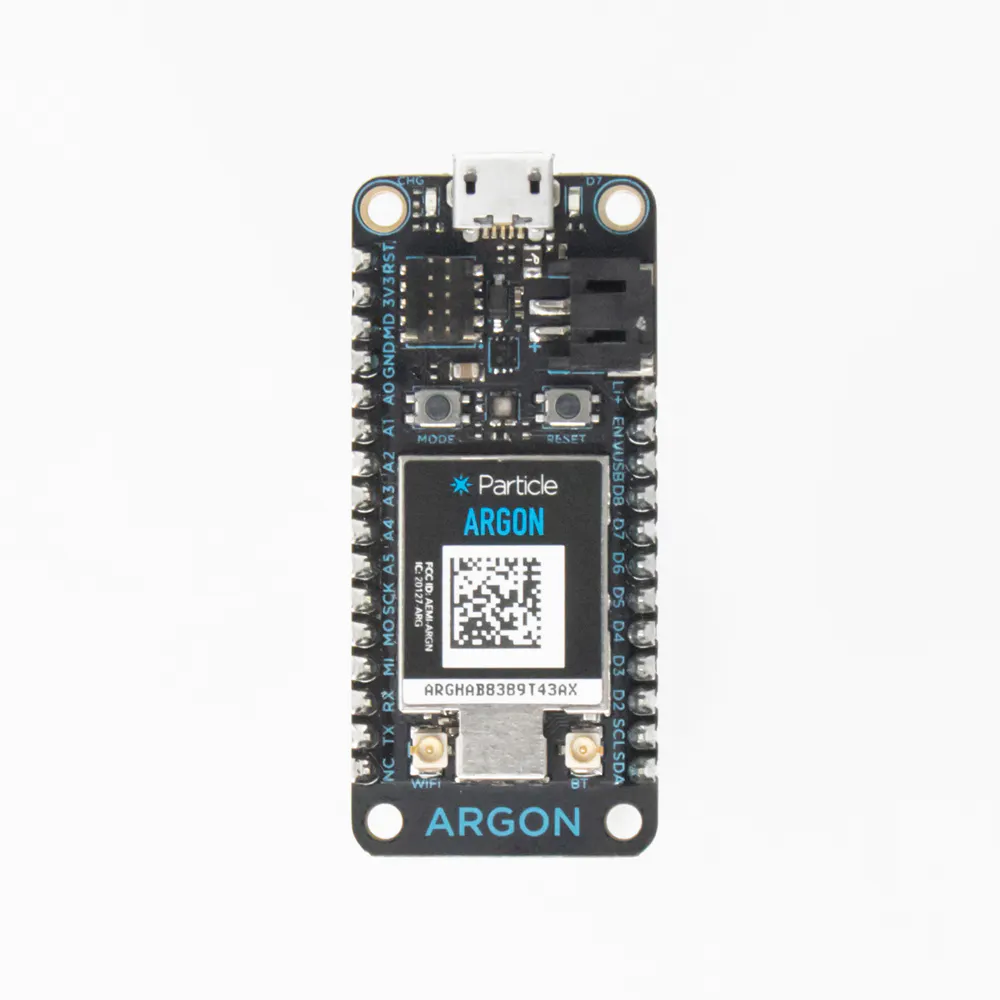Particle Argon
×3×1Jumper wires (generic)
×3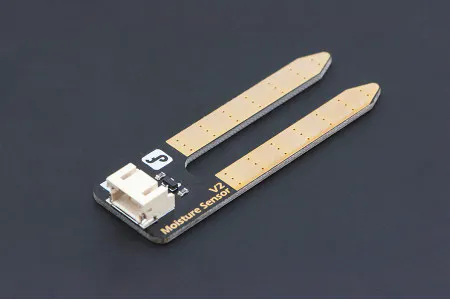DFRobot Gravity: Analog Soil Moisture Sensor For Arduino
×1

### Software apps and online servicesParticle Build Web IDE

## Schematics

### Moisture Circuit Diagram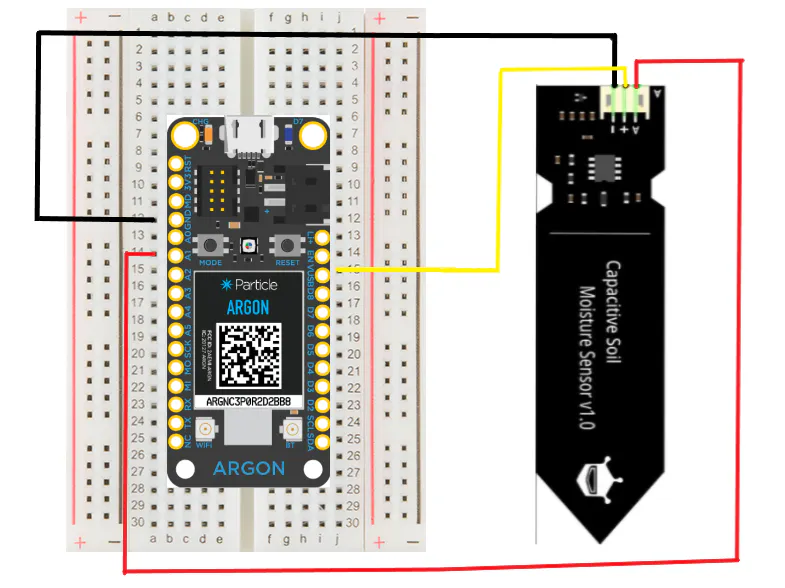### Moisture Level Graph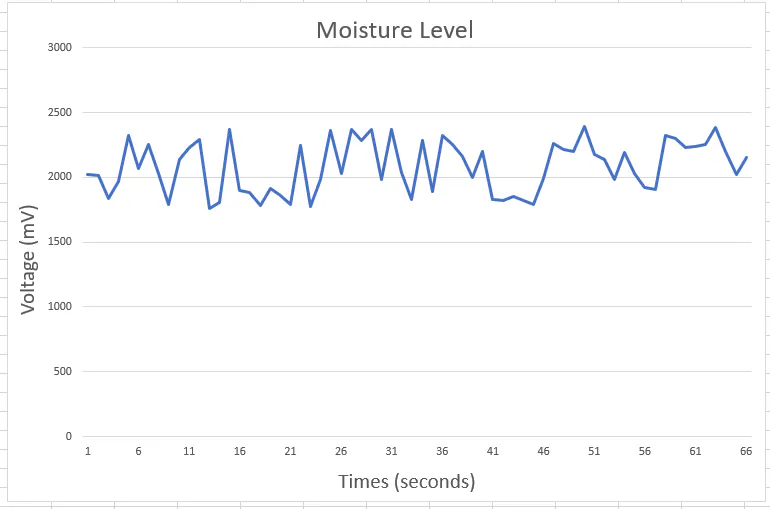## Code

### Argon 1

C/C++
```int i = 0;
void setup() {

pinMode(D7, OUTPUT);
Particle.subscribe("LED", toggleLed, ALL_DEVICES);

if (Particle.connected() == false) {
Particle.connect();
}
Particle.subscribe("need-water", water, ALL_DEVICES);
}
void loop() {
if (digitalRead(D7) == LOW && i < 1) {
delay(5000);
Particle.publish("LED", "on", PUBLIC);
delay(1000);
}
}
String water(const char *event, const char *data) {
return String(data);
}
void toggleLed(const char *event, const char *data) {
if (digitalRead(D7) == LOW && String(data).equals("on")) {
digitalWrite(D7, HIGH);
}
else if (digitalRead(D7) == HIGH && String(data).equals("off")){
digitalWrite(D7, LOW);
}
}
```

### Argon 2

C/C++
```int Moisture = A1;
int analogMoisture = 0;
int mVoltage = 0;
int i = 0;

void setup() {

pinMode(D7, OUTPUT);
Particle.subscribe("LED", toggleLed, ALL_DEVICES);
pinMode(Moisture, INPUT);
}
void loop() {
if (Particle.connected() == false) {
Particle.connect();
}

while (i < 100 && digitalRead(D7) == HIGH) {

MSVoltage = (analogMoistureSoil*3000)/3798;
Particle.variable("MSVoltage", mVoltage);
delay(5000);
}

void toggleLed(const char *event, const char *data) {
if (digitalRead(D7) == LOW && String(data).equals("on")) {
digitalWrite(D7, HIGH);
}
else if (digitalRead(D7) == HIGH && String(data).equals("off")){
digitalWrite(D7, LOW);
}
}
```

### Argon 3

C/C++
```int sum = 0;
int temp = 0;
String value;
int avg = 0;

void setup() {
pinMode(D7, OUTPUT);
Particle.subscribe("LED", toggleLed, ALL_DEVICES);

}

void loop() {

if (Particle.connected() == false) {
Particle.connect();
}
if (j > 40) {
avg = sum / j;
if (avg < 2400 && avg > 0) {
digitalWrite(D7, HIGH);
delay(500);
digitalWrite(D7, LOW);
Particle.publish("need-water", "watered", PUBLIC);
Particle.publish("LED", "off", PUBLIC);
avg = 0;
}
if (avg > 2400) {
digitalWrite(D7, HIGH);
delay(500);
digitalWrite(D7, LOW);
Particle.publish("need-water", "not watered", PUBLIC);
Particle.publish("LED", "off", PUBLIC);
avg = 0;
}
delay(5000);
}

int mVoltage(const char*event, const char*data) {
value = atoi(data);
sum = sum + value.toInt();
return sum;
}
void toggleLed(const char*event, const char*data) {

if (digitalRead(D7) == LOW && String(data).equals("on")) {
digitalWrite(D7, HIGH);
}
else if (digitalRead(D7) == HIGH && String(data).equals("off")){
digitalWrite(D7, LOW);
}
}
```